How to use SUM function in Microsoft Excel

In this article, we will learn why and how we use SUM function in Microsoft Excel.

SUM function is one of the most commonly used “Mathematical” functions in Excel. It adds all the numbers in a range of cells and returns the sum of these values.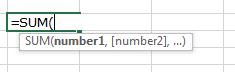With the help of examples, let us understand the use of SUM function in Excel.

Refer to these 5 different examples for the usage of SUM function in Microsoft Excel on the basis of following sample data: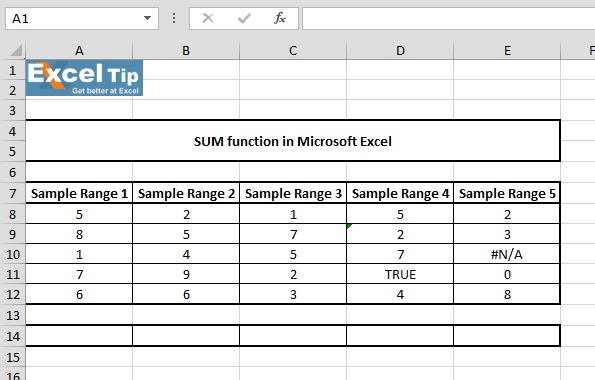1st Example:-

In this example, we will learn how to use SUM function. We have a range of numbers and we want to add all the numbers.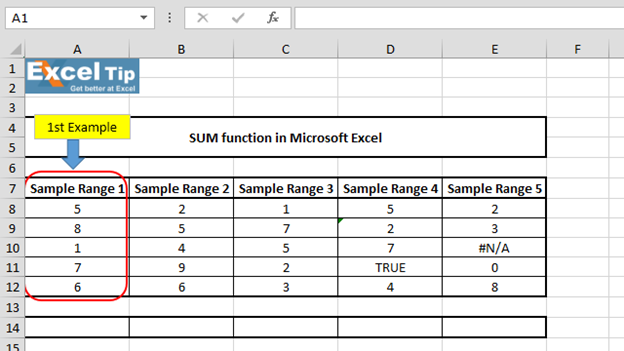Follow the steps given below:-

• Enter the function in cell A14
• =SUM(A8:A12), Press Enter

2nd Example:-
In this example, we’ll first add the values from range and then we’ll add any number to it.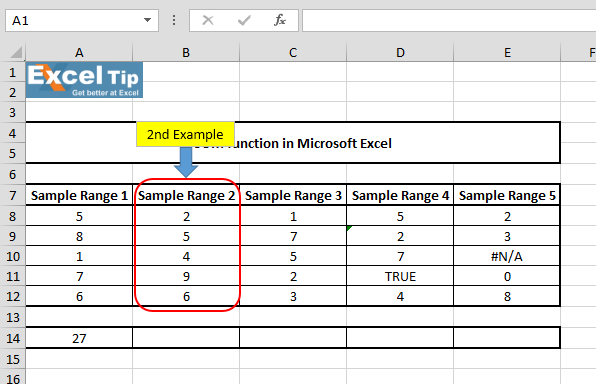Follow the steps given below:-

• Enter the function in cell B14
• =SUM(B8:B12,7), Press Enter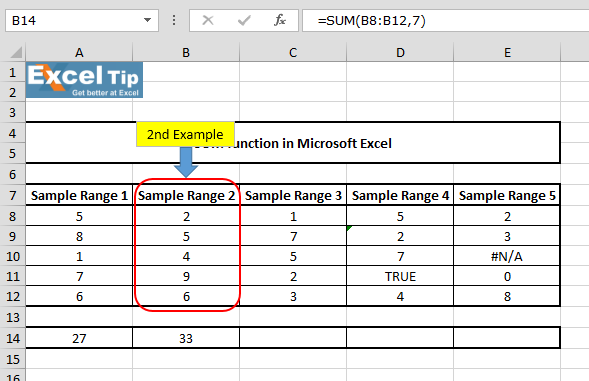It gives us 33 as an answer because function adds the values in cell B8 to B12 first, and then adds 7 to give us 33.

3rd Example:-

In this example, we will return the sum for multiple ranges.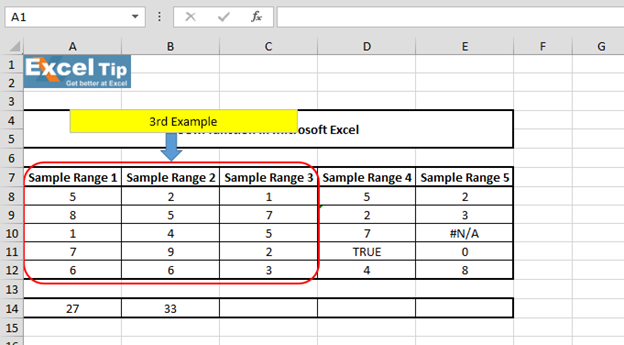Follow the steps given below:-

• Enter the function in cell C14
• =SUM(A8:C12), Press Enter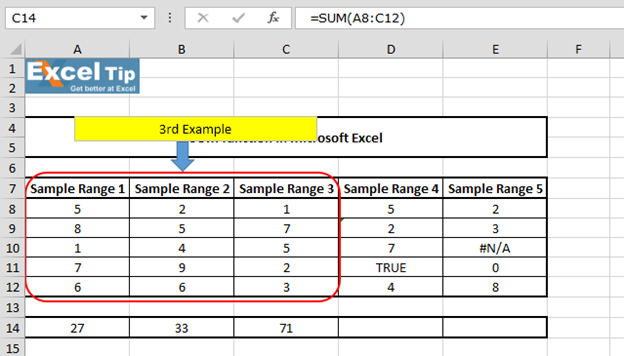The function has returned the sum of 3 different ranges: A8:A12, B8:B12 and C8:C12.

4th Example:-

In this example, we will see what happens when our range contains any logical value.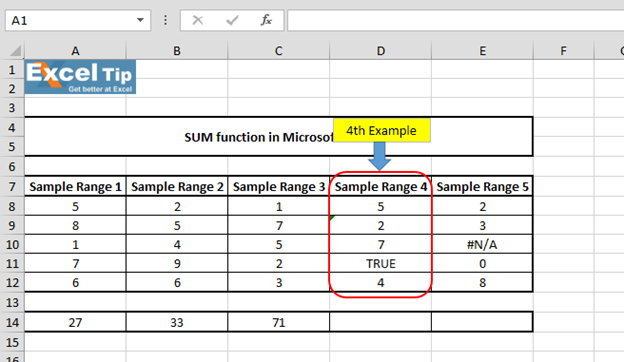Follow the steps given below:-

• Enter the function in cell D14
• =SUM(D8:D12), Press Enter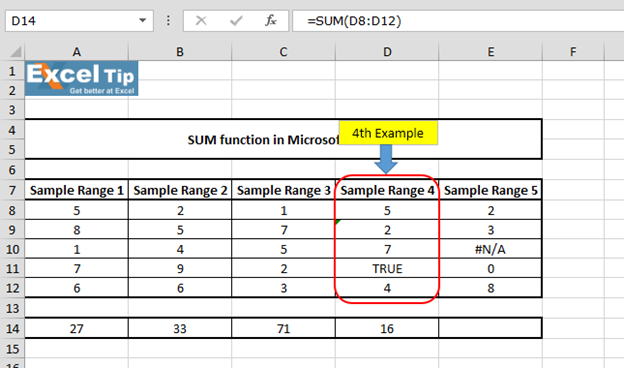The function has returned 16 values. It means SUM function ignores any text value which is comes within specified range.

5th Example:-

In this example, we will see what happens when we enter error value in the range for which we want to calculate sum.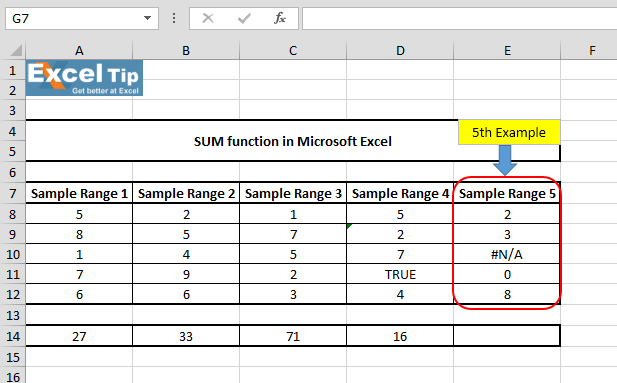Follow the steps given below:-

• Enter the function in cell E14
• =SUM(E8:E12), Press Enter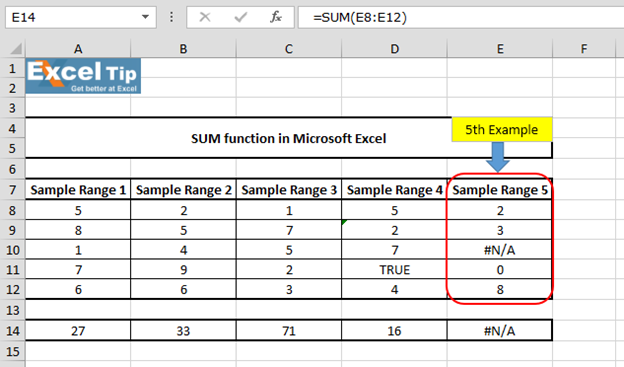It returns #N/A error only. Because, the function does not allow any error value in the range and hence it returns an error.

So, this is how SUM function works in different situations.# Video: How to use SUM function in Microsoft Excel

Click on the video link for quick reference to the use of SUM function. Subscribe to our new channel and keep learning with us!

If you liked our blogs, share it with your friends on Facebook. And also you can follow us on Twitter and Facebook.
We would love to hear from you, do let us know how we can improve, complement or innovate our work and make it better for you. Write us at info@exceltip.com

## Users are saying about us...

1.sanjeevdhiman

how to sum odd no. in a row .but row have an even no. also.

Terms and Conditions of use

The applications/code on this site are distributed as is and without warranties or liability. In no event shall the owner of the copyrights, or the authors of the applications/code be liable for any loss of profit, any problems or any damage resulting from the use or evaluation of the applications/code.